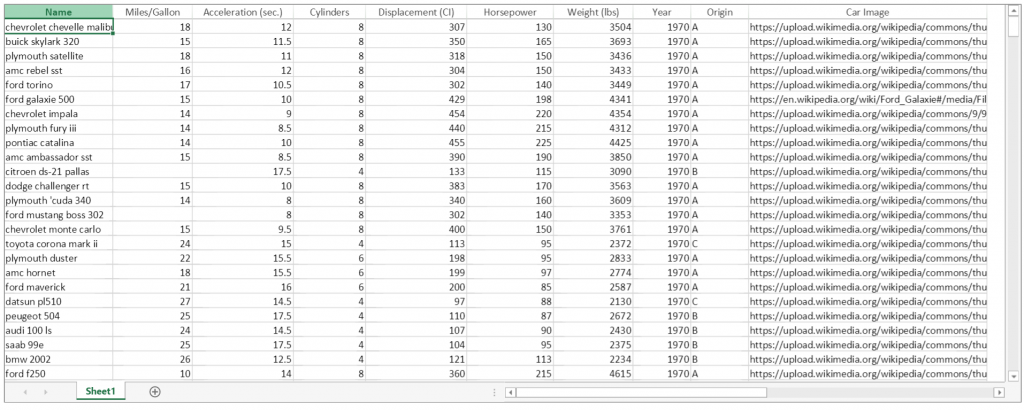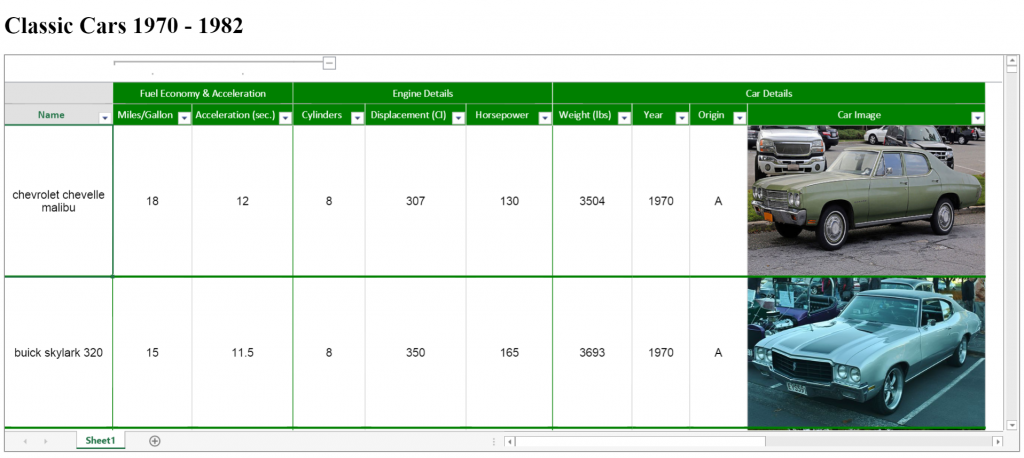### Set Up the Project

Create a new empty ASP.NET Web project, and add a new html file in Visual Studio 2015. In this file, add references to the SpreadJS script and css files, as well as the Angular and jQuery script files:

``````
<!DOCTYPE html>
<html xmlns="http://www.w3.org/1999/xhtml">
<meta charset="utf-8" />

<script src="http://code.jquery.com/jquery-2.1.3.min.js" type="text/javascript"></script>

<script src="Scripts/angular.js" type="text/javascript"></script>
<script src="Scripts/angular-route.js" type="text/javascript"></script>

<body>
</body>
</html>

``````

``````

``````

Once this is done, add a script to the page to contain the Angular code as well as an HTML element that represents the Spread component, setting up the columns that will be bound to data:

``````
<script>
}
</script>
<h1>Classic Cars 1970 - 1982</h1>
<sheets>
<sheet datasource="cars">
<columns>
</columns>
</sheet>
</sheets>
</body>

``````

Before adding code to load the JSON file, create a controller and a module for the Angular application:

``````
<script>
var initialData = null;

});
</script>

``````

Within that controller function, specify a function that will load the data from the JSON file into the “cars” variable for the application:

``````
\$http.get('ClassicCars.json').success(function (data) {
\$scope.cars = data["Classic Cars"];
});
};
});

``````

Going back to the “gcspread-sheets” HTML element that contains the Spread instance on the page, add this controller and initialization function so that the Spread instance is populated with the data from the JSON file:

``````
<h1>Classic Cars 1970 - 1982</h1>
<sheets>
<sheet datasource="cars">
<columns>
//…
</columns>
</sheet>
</sheets>
</body>

``````The data-bound sheet without formatting.

In the controller function for the Spread instance, define the function that formats the SpreadJS component, and apply that formatting to it:

``````
//…

\$http.get('ClassicCars.json').success(function (data) {

for (var i = 0; i < activeSheet.getRowCount() ; i++) {
activeSheet.getColumn(0).wordWrap(true);
activeSheet.getRow(i).font("12pt arial");
activeSheet.setRowHeight(i, 210);
if (activeSheet.getValue(i, 9) != null) {
var carImage = activeSheet.getValue(i, 9);
activeSheet.setValue(i, 9, null);
activeSheet.getCell(i, 9).backgroundImage(carImage);
}
}

var cellRange = new GcSpread.Sheets.Range(0, 0, activeSheet.getRowCount(), 10);
activeSheet.rowFilter(hideRowFilter);

activeSheet.colRangeGroup.group(1, 2);

for (var i = 0; i < activeSheet.getColumnCount() ; i++) {
}

});
};
});

``````

Once the formatting function has been defined, call it when the window is loaded:

``````
If done correctly, the data from the JSON file should show up in the SpreadJS instance on the page, and the component should be formatted like the screenshot below:The data-bound sheet with formatting. In this tutorial, SpreadJS was combined with Angular to implement data binding functionality using an Angular controller and an initialization function. The data was loaded from a JSON file, and the pre-defined column attributes allowed that data to be bound to different columns in the SpreadJS instance. Angular can be combined with SpreadJS to provide an intuitive way to bind data and display it to the user. To learn more about SpreadJS and to download a trial, click here: http://spread.grapecity.com/Downloads/